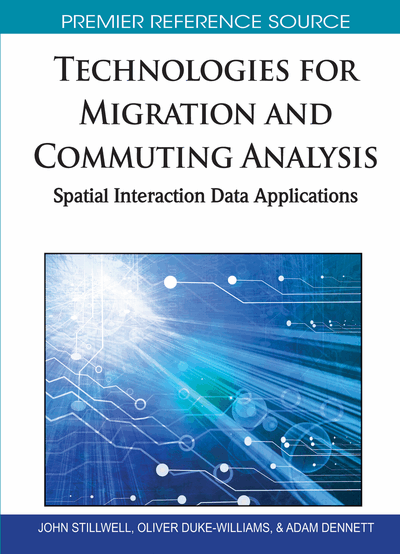# Modelling Migration with Poisson Regression

Robin Flowerdew (University of St. Andrews, UK)
DOI: 10.4018/978-1-61520-755-8.ch014
Available
\$37.50
No Current Special Offers

## Abstract

Most statistical analysis is based on the assumption that error is normally distributed, but many data sets are based on discrete data (the number of migrants from one place to another must be a whole number). Recent developments in statistics have often involved generalising methods so that they can be properly applied to non-normal data. For example, Nelder and Wedderburn (1972) developed the theory of generalised linear modelling, where the dependent or response variable can take a variety of different probability distributions linked in one of several possible ways to a linear predictor, based on a combination of independent or explanatory variables. Several common statistical techniques are special cases of the generalised linear models, including the usual form of regression analysis, Ordinary Least Squares regression, and binomial logit modelling. Another important special case is Poisson regression, which has a Poisson-distributed dependent variable, linked logarithmically to a linear combination of independent variables. Poisson regression may be an appropriate method when the dependent variable is constrained to be a non-negative integer, usually a count of the number of events in certain categories. It assumes that each event is independent of the others, though the probability of an event may be linked to available explanatory variables. This chapter illustrates how Poisson regression can be carried out using the Stata package, proceeding to discuss various problems and issues which may arise in the use of the method. The number of migrants from area i to area j must be a non-negative integer and is likely to vary according to zone population, distance and economic variables. The availability of high-quality migration data through the WICID facility permits detailed analysis at levels from the region to the output areas. A vast range of possible explanatory variables can also be derived from the 2001 Census data. Model results are discussed in terms of the significant explanatory variables, the overall goodness of fit and the big residuals. Comparisons are drawn with other analytic techniques such as OLS regression. The relationship to Wilson’s entropy maximising methods is described, and variants on the method are explained. These include negative binomial regression and zero-censored and zero-truncated models.
Chapter Preview
Top

## Introduction

Poisson regression analysis is a standard but relatively unpublicised (but see Griffith and Haining 2006) statistical technique that is particularly suited to analysis of migration flow data. The Poisson distribution was first identified in 1837 by the French mathematician, Simeon-Denis Poisson (1781-1840). It applies to count data where the variable being analysed must take the form of non-negative integers (i.e. zero or a positive whole number). For large counts, there is little difference between Poisson regression and weighted Ordinary Least Squares (OLS) regression but it does make a difference where some of the counts are small. OLS regression, based on the normal distribution, is the usual form of regression taught in introductory statistics classes. In addition to its theoretical appropriateness, Poisson regression has additional advantages, including ease of constructing multiple regression models and ability to judge model goodness-of-fit.

The classic example of the application of the Poisson model is the distribution of soldiers in corps of the Prussian army who died from mule kicks. Counts of deaths were available for 10 of 14 corps for each of 20 years (Griffith and Haining, 2006). These deaths were fairly rare and independent of each other, so it was appropriate to investigate if the data followed the Poisson distribution. Whilst this example is rather unusual, essentially any count data can be modelled as Poisson provided that it can be regarded as a total of events of any kind that occur within a time period. For example, the number of people contracting a rare disease, the number of cars passing a checkpoint or the number of convicted criminals coming from different areas, are all possible data sets for Poisson regression. Senior (1987) has used it to study the number of family planning clinics in a set of Nigerian cities. Guy (1991) has used it for analysis of retailing data. The most frequent use of Poisson models is in the analysis of contingency tables. The counts recorded can be modelled as functions of the main effects of each cross-classifying variable and interaction effects involving these variables in any combination. The interest is in determining which interactions are significant and which are not. This is a special case of Poisson regression but it is the case which has received most attention in the statistics literature, from Nelder and Wedderburn (1972) onwards.

Flowerdew and Aitkin (1982) introduced Poisson regression in the context of migration analysis, and Flowerdew (1991) provided an updated account of Poisson models of migration, including comparisons with other modelling strategies. Lovett and Flowerdew (1989) published a pedagogic account of Poisson models in geography. Poisson models are not discussed in much detail in texts presenting statistical techniques to geographers, although Bailey and Gatrell (1995) and Haining (2003) do deal with them briefly, and O’Brien (1992) in a bit more detail. Similarly, discussions in statistics or econometrics texts are relatively few, exceptions including Greene (1999), Kirkwood and Stern (2005, Chapter 24) and Petrie and Sabin (2005, Chapter 31). The fullest account of Poisson regression and its variants is that by Cameron and Trivedi (1998).

This chapter is intended to present Poisson regression and some of its variants as a suitable method for analysing migration flows. The argument is illustrated through an analysis of inter-district migration in Great Britain from the 2001 Census. It shows how a Poisson regression model can be fitted to such data, and discusses issues which may arise in the process, including the use of regression models based on other count distributions such as the negative binomial.

## Complete Chapter List

Search this Book:
Reset